Do you like the article?
Share it with others —
post a link to it!
Use new possibilities of MetaTrader 5

#### Similar articles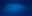# Learn how to design a trading system by Bear's Power

MetaTrader 5Trading | 10 August 2022, 16:1010 9670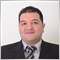### Introduction

In this new article from our series, we will learn a new technical tool that can be used in our favor especially if we combine it with other technical tools. We will learn how to create a trading system by the Bear's Power technical indicator. As we do in our article in this series that we try to understand the root of things to use them in an effective way. We learn about the Bear's Power indicator in detail through the following topics:

Through the topic of Bear's Power definition, we will learn in detail about the Bear's Power indicator through learning what it is, what it measures, how we can calculate it manually to learn the basic concept behind this indicator, and how we can insert and use it in the MetaTrader 5 trading platform. After learning the basics and understanding the main concept behind this indicator we will learn how to use it through some simple strategies to deepen our understanding and to get more insights about this topic of the article to improve our trading and this will be learned through the topic of Bear's Power strategy. Then, we will design a step-by-step blueprint to create a trading system based on mentioned strategies and this step is very important as it will help us to create our trading smoothly the same we will see in the topic of Bear's Power strategy blueprint. After that, we will come to the most interesting topic in this article which is Bear's Power trading system because we will learn how to create trading based on mentioned strategies by MQL5 (MetaQuotes Language) to be executed in the MetaTrader 5 trading terminal.

I advise trying to apply and code what you learn by yourself as practicing is an important factor in any learning process as this will help you to understand the topic very well. We will use this article as I mentioned the MetaTrader 5 trading platform and its built-in IDE to write our MQL5 codes. If you want to know how you can download and use them you can read the topic of Writing MQL5 code in MetaEditor from a previous article.

Disclaimer: All information provided 'as is' only for educational purposes and is not prepared for trading purposes or advice. The information does not guarantee any kind of result. If you choose to use these materials on any of your trading accounts, you will do that at your own risk and you will be the only responsible.

Now, let us start our topics.

### Bear's Power definition

In this topic, we will learn in more detail about the Bear's Power indicator through learning what it is, what it measures, and how we can calculate and use it in the MetaTrader 5. Bear's Power indicator is an oscillator indicator that oscillates around zero level and it measures the bearishness in the market it can also give us an indication that bulls come into the game and that when we see that bears became weak. We all recognize that supply and demand are very important in any marketplace as it is vehicles and power that are moving markets up or down. So, it is important to know how much bulls and bears are controlling markets. This indicator is created by Dr. Alexander Elder to measure this concept and see how much bears are controlling the market.

How this indicator can do that, this question we can get the answer to if we learned how we can calculate it. To calculate this Bear's Power indicator we will follow the following.

1. Get the low of the period.
2. Get the exponential moving average(EMA), to learn more about it and how we can calculate it, you can do that by reading my previous article about the moving average, Learn how to design different moving average systems.
3. Get the Bear's Power value by subtracting the EMA from the low.

Low = the lowest values during the period.

EMA = the exponential moving average EMA.

Bear's Power = Low - EMA

We know that bears control the market, they keep pushing the market lower most of the time. So, we use the low's value in the formula of Bear's Power calculation as we need to measure these bears then we will get the difference between this low and the EMA to get an oscillator indicator that oscillates around zero level and when we find that its value approaches the zero level and became higher than it we can get an indication that bears became weaker.

It is good to use this indicator with another trending indicator as it will give more effective insights the same as we will do in one mentioned strategies in this article. This concept is very magnificent and one of the beautiful features of technical analysis as we can use many concepts to get more insights and see the instrument from different perspectives.

If we want to know how we can insert this indicator to the MetaTrader 5 we can do that while opening the trading terminal by pressing Insert --> Indicators --> Oscillators --> Bears Power. We can see that also through the following picture: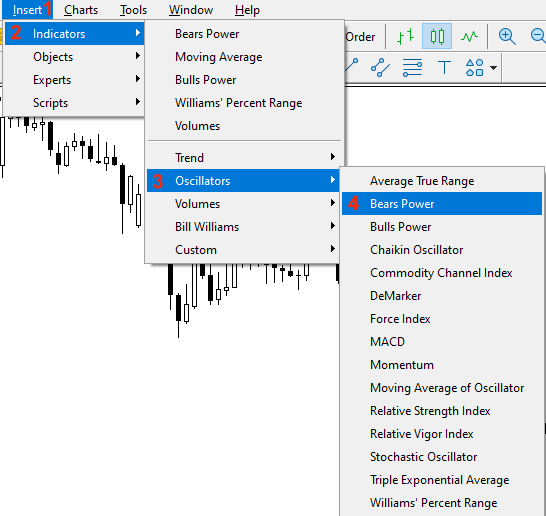After pressing the "Bears Power" to insert it on the chart we will find the window of its parameters the same as the following: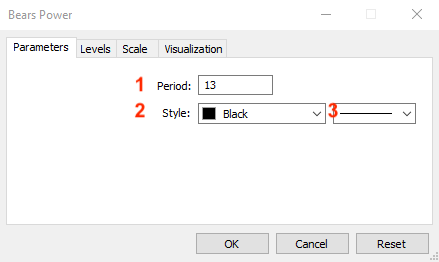1 - To determine the period that will be used in the calculation.

2 - To determine the color of bars of Bear's Power.

3 - To determine the thickness of bars of Bear's Power.

After determining these parameters and pressing "OK", we will find that the indicator is inserted into the chart the same as the following: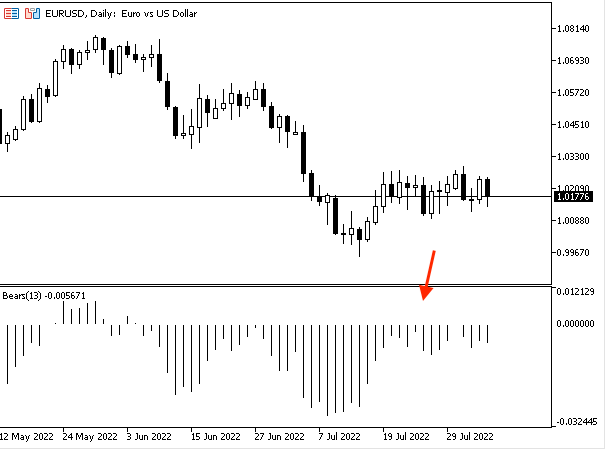As we can in the previous chart in the lower part we have the indicator attached to the chart and its bars oscillate around zero the same as we mentioned when we find the value below zero it means that bears control and it approaches zero and became higher than it this means that they became weaker.

### Bear's Power strategy

In this part, we will learn how we can use Bear's Power through simple strategies that can be used based on the basic concept of this indicator. The following are for these strategies and their conditions. I need to confirm here, that these strategies for education as the main objective is to understand the main concept behind the indicator and how we can use it, so you must test any of them before using them on your real account to make sure that it will be good for your trading.

Strategy one: Bear's Power Movement

According to this strategy, we need to get signals based on the position of current and previous bear's power values. If the current value is greater than the previous, this will be a signal of the rising of Bear's Power indicator. Vice versa, if the current value is lower than the previous value, this will be a signal of declining Bear's Power.

Simply,

Current Bear's Power > Previous Bear's Power --> Bear's Power is Rising

Current Bear's Power < Previous Bear's Power --> Bear's Power is declining

Strategy two: Bear's Power - Strong or Divergence

According to this strategy, we need to get a signal that informs us if there are strong movements or there are divergences by evaluating four values and they are current low, previous low, bear power, and previous bear power. If the current low is lower than the previous low and the current bear power value is lower than the previous one, this will be a signal of a strong move. In the other case, if the current low is lower than the previous low and the current bear value is greater than the previous one, this will be a signal of bullish divergence.

Simply,

Current low < previous low and current bear's power < previous bear's power --> strong move

Current low < previous low and current bear's power > previous bear's power --> bullish divergence

Strategy three

According to this strategy, we need a trigger that can be used to get buy and sell signals and we will evaluate four values to do that based on this strategy. These four values are current bear's power, zero level, current close value, and current exponential moving average. If the current bear's power is greater than the zero level and the current close is greater than the exponential moving average, this will be a signal of buy. If the current bear's power is lower than the zero level and the current close is lower than the exponential moving average, this will be a signal of selling.

Simply,

Current bear's power > zero level and current close > EMA --> buy

Current bear's power < zero level and current close < EMA --> sell

### Bear's Power strategy blueprint

In this topic, we will learn how to design a step-by-step blueprint for each mentioned strategy to help us to create trading systems for them smoothly and easily after organizing our ideas in clear steps in this blueprint.

Strategy one: Bear's Power Movement

First, we need to understand what we need the program to do to organize our steps, so, we need the computer to check two values every tick, and that will be after creating these values for sure, these values are current Bear's Power and previous Bear's Power. We need the program to check these values to decide which one is greater than the other. If the current one is greater than the previous, we need the program or the expert to return a signal of comment on the chart with the following:

• Bear's Power is rising
• Bear's Power Value
• Bear's Power Previous Value

We need to appear every value in a separate line.

The other scenario is if the current Bear's Power value is greater than the previous one, we need the expert advisor to return a comment also with the following values and each value in a separate line:

• Bear's Power is declining
• Bear's Power Value
• Bear's Power Previous Value.

So, The following is a step-by-step blueprint for this strategy to create a trading system.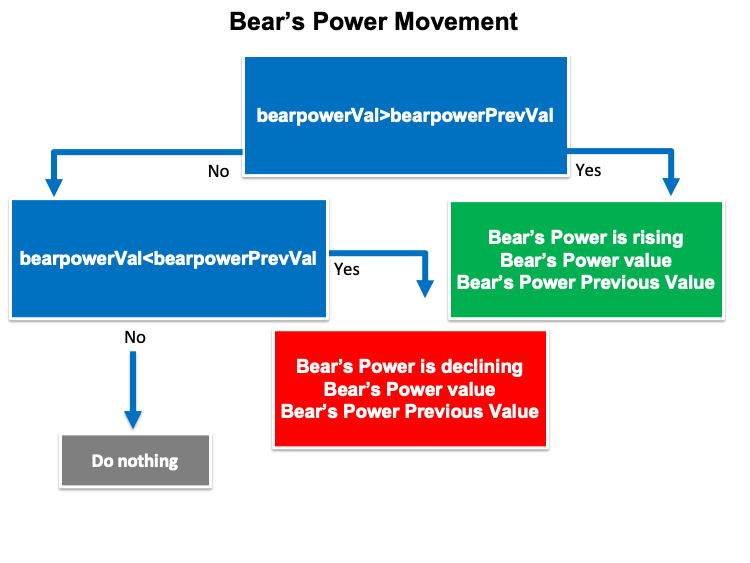Strategy two: Bear's Power - Strong or Divergence

Based on this strategy, we need the trading system to check four values and they are current low, previous low, current bear power, and previous bear power values. After that we need to decide if the current low value is lower than the previous one and at the same time the current bear's power value is lower than the previous one, we need the trading system to return a signal as a comment on the chart with the following values and each one of them will be in a separate line:

• Strong Move
• Current Low Value
• Previous Low Value
• Current Bear's Power Value
• Previous Bear's Power Value

In the other case, if the current low value is lower than the previous one and at the same time, the current bear's power value is greater than the previous one, we need the trading system to return a signal of comment on the chart with the following values:

• Bullish divergence
• Current Low Value
• Previous Low Value
• Current Bear's Power Value
• Previous Bear's Power Value

The following is a step-by-step blueprint to help us to create a trading system based on this strategy.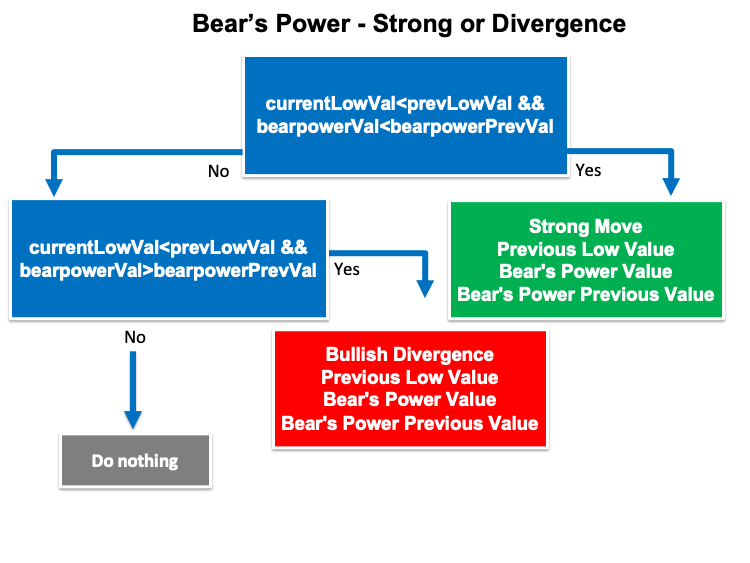Strategy three: Bear's Power signals

Based on this strategy, we need to create a trading system that checks four values every tick and they are the current bear's power, zero level, the current close, and the current exponential moving average. We need to decide if the current bear's power is greater than the zero level and at the same time if the current close is greater than the exponential moving average, we need the expert advisor to return a signal of comment on the chart with the following values and each one of them in a separate line:

• Current Close Value
• Current EMA Value
• Current Bear's Power Value

In the other case, if the current bear's power is lower than zero and at the same time, the current close is lower than the exponential moving average, we need the trading system to return a signal of comment with the following values:

• Sell Signal
• Current Close Value
• Current EMA Value
• Current Bear's Power Value

The following is a step-by-step blueprint to organize our ideas to create a trading system based on this strategy.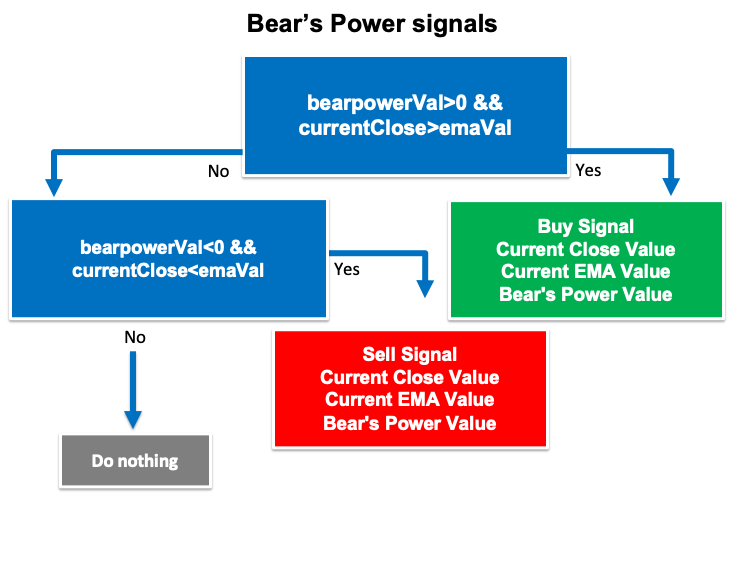### Bear's Power trading system

In this topic, we will learn how to create a trading system for each mentioned strategy but we will create a simple trading system for Bear's power to return a signal of comment on the chart with the current value of Bear's power to use it as a base for all strategies.

The following is for the code to create this trading system.

We will create an array for the "bearpower" by using the double function:

`double bearpowerArray[];`

Sorting this created array by using the "ArraySetAsSeries" function to return a boolean value.

`ArraySetAsSeries(bearpowerArray,true);`

Defining the Bear's Power indicator by using the "iBearsPower" function to return the handle of the indicator. Parameters are:

• symbol: we will use (_Symbol) to be applied on the current symbol.
• period: we will use (_Period) to be applied on the current period or timeframe.
• ma_period: we will use 13 for the period of usable moving average.
`int bearpowerDef = iBearsPower(_Symbol,_Period,13);`

Fill the array by using the CopyBuffer function to get the data from the Bear's Power indicator. Parameters of this function:

• indicator_handle: for indicator handle and we will use (bearpowerDef).
• buffer_num: for indicator buffer number and we will use (0).
• start_pos: for start position and we will use (0).
• count: for the amount to copy and we will use (3).
• buffer[]: for the target array to copy and we will use (bearpowerArray).
`CopyBuffer(bearpowerDef,0,0,3,bearpowerArray);`

Defining the "bearpowerVal" after creating a variable by using the "NormalizeDouble" function to return the double type value. Parameters of this function:

• value: we will use (bearpowerArray) as a normalized number.
• digits: we will use (6) as a number of digits after the decimal point.
`double bearpowerVal = NormalizeDouble(bearpowerArray,6);`

We need to generate a comment on the chart with the current Bear's Power value by using the "Comment" function:

`Comment("Bear's Power Value is ",bearpowerVal);`

The following is the full code to create this trading system:

```//+------------------------------------------------------------------+
//|                                          Simple Bear's Power.mq5 |
//|                                  Copyright 2022, MetaQuotes Ltd. |
//|                                             https://www.mql5.com |
//+------------------------------------------------------------------+
#property version   "1.00"
//+------------------------------------------------------------------+
void OnTick()
{
double bearpowerArray[];

ArraySetAsSeries(bearpowerArray,true);

int bearpowerDef = iBearsPower(_Symbol,_Period,13);

CopyBuffer(bearpowerDef,0,0,3,bearpowerArray);

double bearpowerVal = NormalizeDouble(bearpowerArray,6);

Comment("Bear's Power Value is ",bearpowerVal);

}
//+------------------------------------------------------------------+```

After compiling this code we will find the expert of this trading system in the navigator window the same as the following: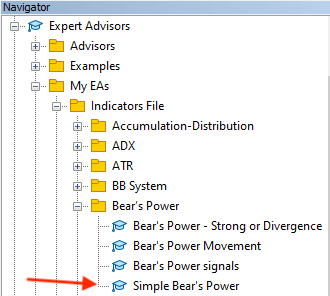By dragging and dropping it on the chart we will find that the window of this expert will appear the same as the following: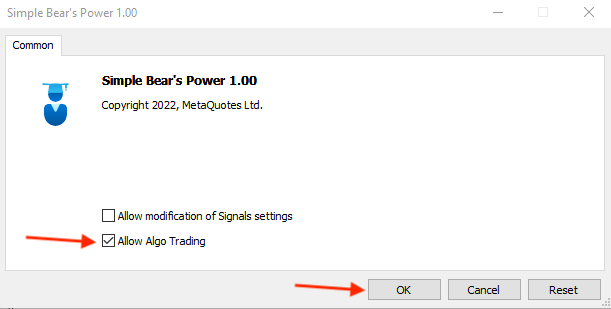After pressing "OK" we will find the expert is attached to the chart the same as the following: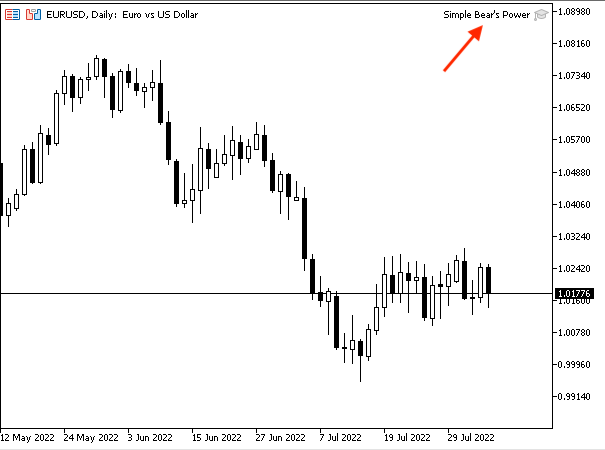As we can see in the previous chart in the top right corner that the expert is attached. Then, we can find generated signals on the chart the same as the following as an example from testing: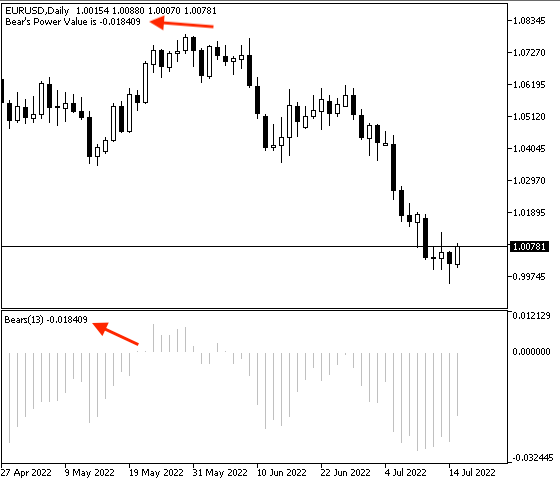As we can see in the previous chart in the top left corner that we have the current Bear's Power value.

Strategy one: Bear's Power Movement

The following is the full code to create a trading system for this strategy:

```//+------------------------------------------------------------------+
//|                                        Bear's Power Movement.mq5 |
//|                                  Copyright 2022, MetaQuotes Ltd. |
//|                                             https://www.mql5.com |
//+------------------------------------------------------------------+
#property version   "1.00"
//+------------------------------------------------------------------+
void OnTick()
{
double bearpowerArray[];

ArraySetAsSeries(bearpowerArray,true);

int bearpowerDef = iBearsPower(_Symbol,_Period,13);

CopyBuffer(bearpowerDef,0,0,3,bearpowerArray);

double bearpowerVal = NormalizeDouble(bearpowerArray,6);
double bearpowerPrevVal = NormalizeDouble(bearpowerArray,6);

if(bearpowerVal>bearpowerPrevVal)
{
Comment("Bear's Power is rising","\n",
"Bear's Power Value is ",bearpowerVal,"\n",
"Bear's Power Previous Value is ",bearpowerPrevVal);
}

if(bearpowerVal<bearpowerPrevVal)
{
Comment("Bear's Power is declining","\n",
"Bear's Power Value is ",bearpowerVal,"\n",
"Bear's Power Previous Value is ",bearpowerPrevVal);
}
}
//+------------------------------------------------------------------+```

Differences in this code:

Defining the current bear's power value "bearpowerVal" and the previous bear's power value "bearpowerPrevVal":

```   double bearpowerVal = NormalizeDouble(bearpowerArray,6);
double bearpowerPrevVal = NormalizeDouble(bearpowerArray,6);```

Conditions of the strategy:

In case of rising:

```   if(bearpowerVal>bearpowerPrevVal)
{
Comment("Bear's Power is rising","\n",
"Bear's Power Value is ",bearpowerVal,"\n",
"Bear's Power Previous Value is ",bearpowerPrevVal);
}```

In case of declining:

```   if(bearpowerVal<bearpowerPrevVal)
{
Comment("Bear's Power is declining","\n",
"Bear's Power Value is ",bearpowerVal,"\n",
"Bear's Power Previous Value is ",bearpowerPrevVal);
}```

After compiling this code we can find the expert of this trading system in the navigator window the same as the following: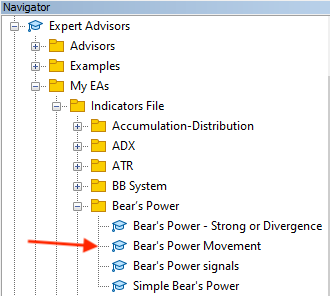By dragging and dropping the expert on the chart we will find the window of this expert the same as the following:After pressing "OK" we will find that it will be attached to the chart the same as the following: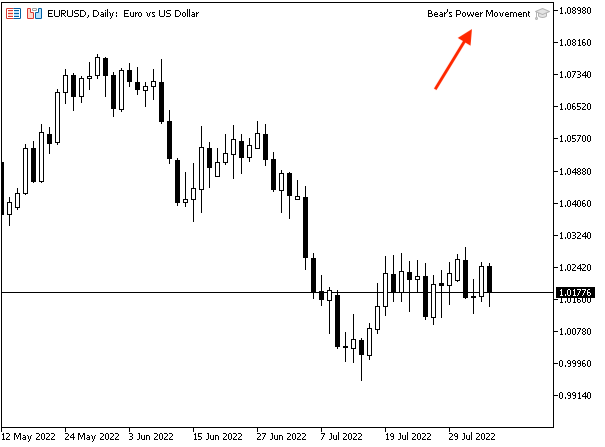As we can see on the previous chart in the top right corner that the expert is attached to the chart. Then, we can find generated signals the same as the following as per the signal.

In case of rising with current data: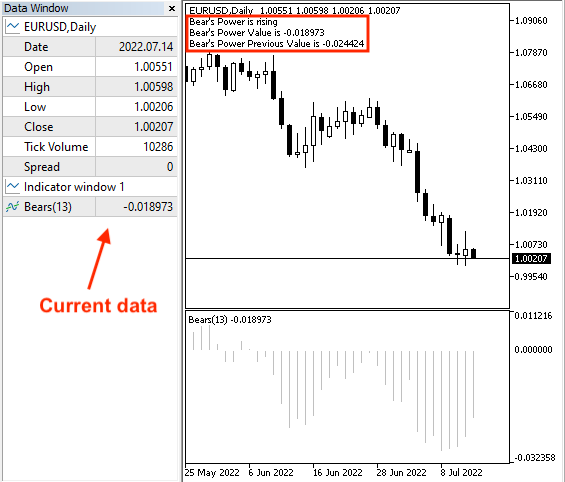As we can see on the previous chart in the upper part of the chart, we have generated a rising signal, the current bear's power value, and the previous bear's power value. In the data window, we can find the current value of the bear's power.

In case of rising with previous data: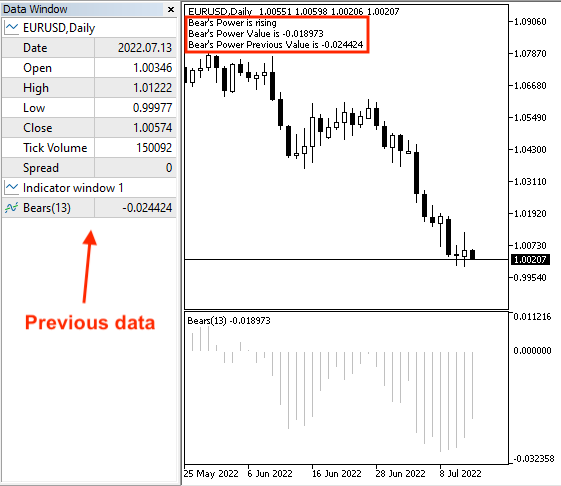As we can see on the previous chart, we can find a difference in the data window as we can find the previous value of the bear's power.

In case of declining with current data: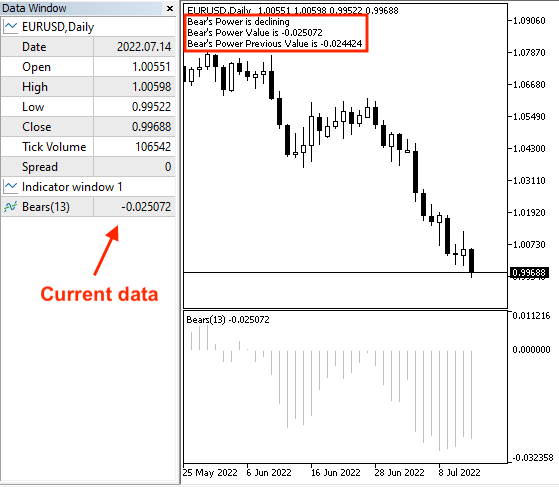As we can see on the previous chart in the upper part of the chart, we have generated a declining signal, the current bear's power value, and the previous bear's power value. In the data window, we can find the current value of the bear's power.

In case of declining with previous data: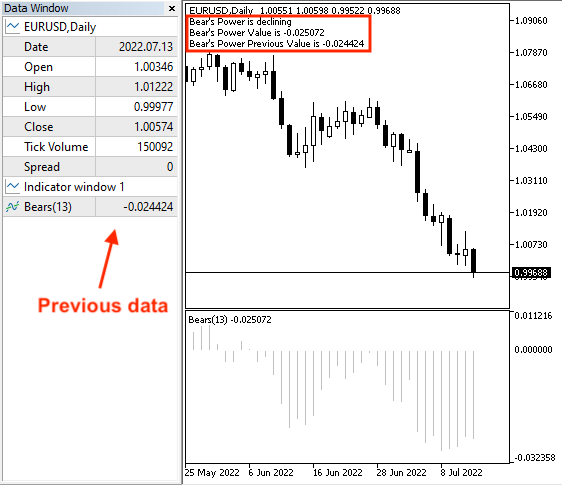As we can see on the previous chart, we can find a difference in the data window as we can find the last value of the bear's power.

Strategy two: Bear's Power - Strong or Divergence

The following is the full code to create a trading system for this strategy:

```//+------------------------------------------------------------------+
//|                          Bear's Power - Strong or Divergence.mq5 |
//|                                  Copyright 2022, MetaQuotes Ltd. |
//|                                             https://www.mql5.com |
//+------------------------------------------------------------------+
#property version   "1.00"
//+------------------------------------------------------------------+
void OnTick()
{
double bearpowerArray[];
MqlRates priceArray[];

ArraySetAsSeries(bearpowerArray,true);
int Data=CopyRates(_Symbol,_Period,0,3,priceArray);

int bearpowerDef = iBearsPower(_Symbol,_Period,13);

CopyBuffer(bearpowerDef,0,0,3,bearpowerArray);

double bearpowerVal = NormalizeDouble(bearpowerArray,6);
double bearpowerPrevVal = NormalizeDouble(bearpowerArray,6);

double currentLowVal=NormalizeDouble(priceArray.low,6);
double prevLowVal=NormalizeDouble(priceArray.low,6);

if(currentLowVal<prevLowVal && bearpowerVal<bearpowerPrevVal)
{
Comment("Strong Move","\n",
"Current Low Value is ",currentLowVal,"\n",
"Previous Low Value is ",prevLowVal,"\n",
"Bear's Power Value is ",bearpowerVal,"\n",
"Bear's Power Previous Value is ",bearpowerPrevVal);
}

if(currentLowVal<prevLowVal && bearpowerVal>bearpowerPrevVal)
{
Comment("Bullish divergence","\n",
"Current Low Value is ",currentLowVal,"\n",
"Previous Low Value is ",prevLowVal,"\n",
"Bear's Power Value is ",bearpowerVal,"\n",
"Bear's Power Previous Value is ",bearpowerPrevVal);
}
}
//+------------------------------------------------------------------+```

Differences in this code:

Creating two arrays, bearpowerArray and priceArray:

```   double bearpowerArray[];
MqlRates priceArray[];```

Sorting these created arrays, for the bearpowerArray we will use using the "ArraySetAsSeries" function the same as we learned before. For the priceArray, we will use the "CopyRates" function to get historical data of "MqlRates" and its parameters are:

• symbol name: we will use (_Symbol).
• timeframe: we will use (_Period).
• start time: we will use (0).
• stop time: we will use (3).
• rates array: we will use (pArray).
```   ArraySetAsSeries(bearpowerArray,true);
int Data=CopyRates(_Symbol,_Period,0,3,priceArray);```

Defining current and previous values of bear's power and lows by using the "NormalizeDouble" function.

```   double bearpowerVal = NormalizeDouble(bearpowerArray,6);
double bearpowerPrevVal = NormalizeDouble(bearpowerArray,6);

double currentLowVal=NormalizeDouble(priceArray.low,6);
double prevLowVal=NormalizeDouble(priceArray.low,6);```

Conditions of strategy:

In case of strong move:

```   if(currentLowVal<prevLowVal && bearpowerVal<bearpowerPrevVal)
{
Comment("Strong Move","\n",
"Current Low Value is ",currentLowVal,"\n",
"Previous Low Value is ",prevLowVal,"\n",
"Bear's Power Value is ",bearpowerVal,"\n",
"Bear's Power Previous Value is ",bearpowerPrevVal);
}```

In case of bullish divergence:

```   if(currentLowVal<prevLowVal && bearpowerVal>bearpowerPrevVal)
{
Comment("Bullish divergence","\n",
"Current Low Value is ",currentLowVal,"\n",
"Previous Low Value is ",prevLowVal,"\n",
"Bear's Power Value is ",bearpowerVal,"\n",
"Bear's Power Previous Value is ",bearpowerPrevVal);
}```

After compiling this code we can find the expert of this trading system in the navigator window: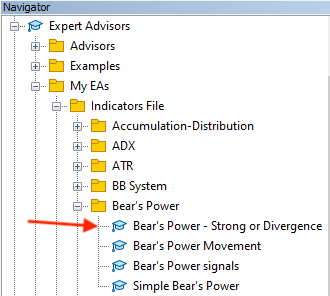By dragging and dropping this expert on the desired chart, we will find the window of it the same as the following: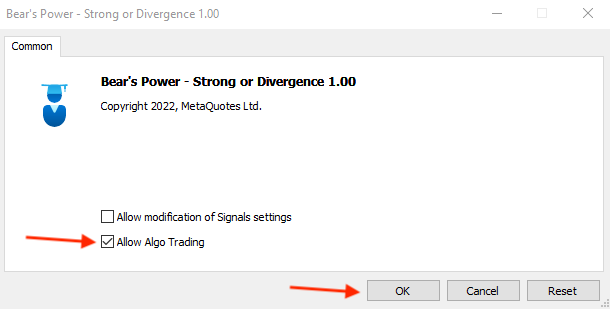After pressing "OK" we will find that the expert is attached the same as the following: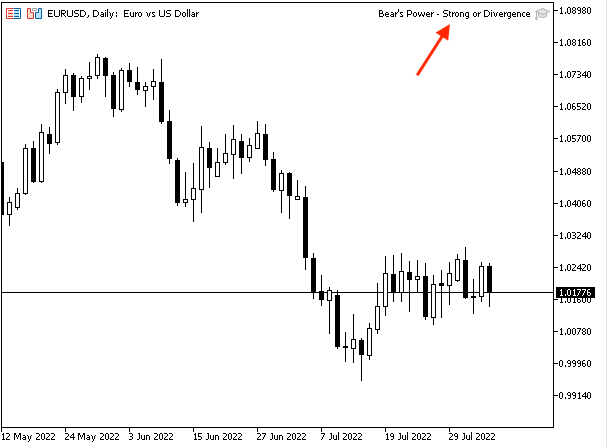As we can see on the previous chart, in the top right corner of the chart the expert of Bear's Power - Strong or Divergence is attached to the chart. Then, we can find generated signals based on this strategy the same as the following.

In case of a strong move with current data: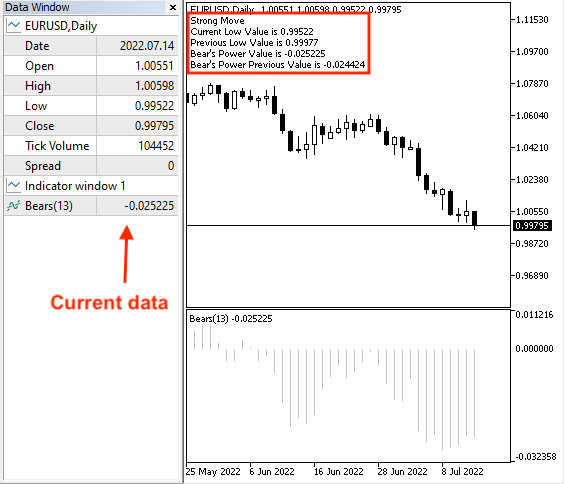As we can see on the chart we have the desired signal the same as we want with a comment of the following values:

• Strong move
• Current low value
• Previous low value
• Current Bear's Power value
• Previous Bear's Power value

In case of strong with previous data: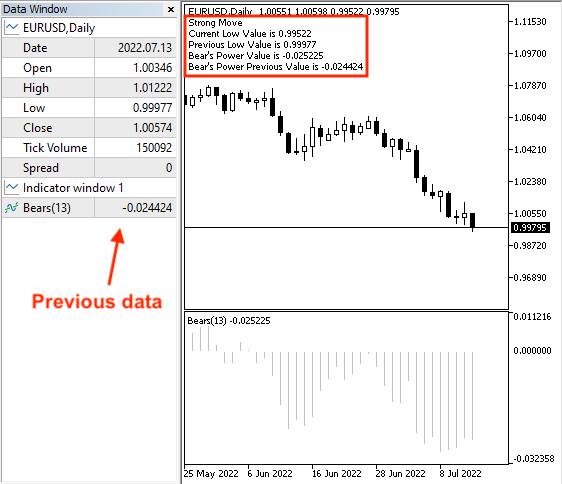As we can see on the previous chart we have the same comment as a signal on the chart with the same values but with the previous data window.

In case of bullish divergence with current data:As we can see on the previous chart we have a comment on the chart as a comment with the following values:

• Bullish Divergence
• Current low value
• Previous low value
• Current Bear's Power value
• Previous Bear's Power value

In case of bullish divergence with previous data: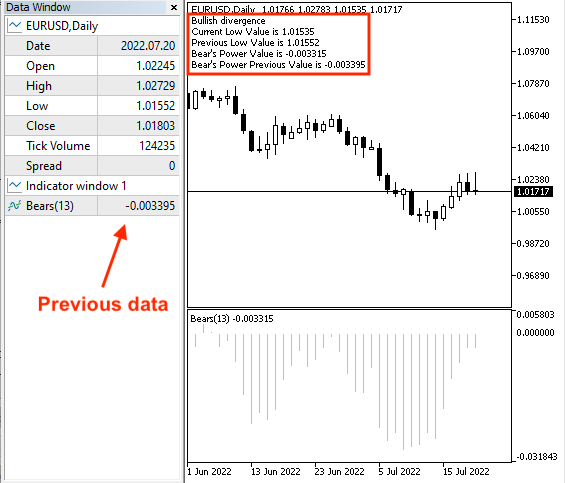As we can see on the previous chart we have a comment on the chart with the same value as the previous data window.

Strategy three: Bear's Power signals

The following is the full code to create a trading system based on this strategy.

```//+------------------------------------------------------------------+
//|                                         Bear's Power signals.mq5 |
//|                                  Copyright 2022, MetaQuotes Ltd. |
//|                                             https://www.mql5.com |
//+------------------------------------------------------------------+
#property version   "1.00"
//+------------------------------------------------------------------+
void OnTick()
{
double bearpowerArray[];
double maArray[];
MqlRates priceArray[];

ArraySetAsSeries(bearpowerArray,true);
ArraySetAsSeries(maArray,true);
int Data=CopyRates(_Symbol,_Period,0,3,priceArray);

int bearpowerDef = iBearsPower(_Symbol,_Period,13);
int maDef = iMA(_Symbol,_Period,13,0,MODE_EMA,PRICE_CLOSE);

CopyBuffer(bearpowerDef,0,0,3,bearpowerArray);

double bearpowerVal = NormalizeDouble(bearpowerArray,6);

double emaVal = NormalizeDouble(maArray,6);

double currentClose=NormalizeDouble(priceArray.close,6);

if(bearpowerVal>0 && currentClose>emaVal)
{
"Current Close Value is ",currentClose,"\n",
"Current EMA Value is ",emaVal,"\n",
"Bear's Power Value is ",bearpowerVal);
}

if(bearpowerVal<0 && currentClose<emaVal)
{
Comment("Sell Signal","\n",
"Current Close Value is ",currentClose,"\n",
"Current EMA Value is ",emaVal,"\n",
"Bear's Power Value is ",bearpowerVal);
}
}
//+------------------------------------------------------------------+
```

Differences in this code:

Creating three arrays, bearpowerArray, naArray, and priceArray.

```   double bearpowerArray[];
double maArray[];
MqlRates priceArray[];```

Sorting these created arrays.

```   ArraySetAsSeries(bearpowerArray,true);
ArraySetAsSeries(maArray,true);
int Data=CopyRates(_Symbol,_Period,0,3,priceArray);```

Defining the Bear's Power indicator by using the "iBearsPower" the same as we mentioned before and Moving Average indicator by using the "iMA" function that returns the handle of the moving average indicator.

```   int bearpowerDef = iBearsPower(_Symbol,_Period,13);
int maDef = iMA(_Symbol,_Period,13,0,MODE_EMA,PRICE_CLOSE);```

Fill the array by using the "CopyBuffer" function to get the data from the Bear's Power and moving average indicators.

```   CopyBuffer(bearpowerDef,0,0,3,bearpowerArray);

Defining current bear's power, exponential moving average, and current closing price.

```   double bearpowerVal = NormalizeDouble(bearpowerArray,6);
double emaVal = NormalizeDouble(maArray,6);
double currentClose=NormalizeDouble(priceArray.close,6);```

Conditions of this strategy:

In case of the buy signal:

```   if(bearpowerVal>0 && currentClose>emaVal)
{
"Current Close Value is ",currentClose,"\n",
"Current EMA Value is ",emaVal,"\n",
"Bear's Power Value is ",bearpowerVal);
}```

In case of the sell signal:

```   if(bearpowerVal<0 && currentClose<emaVal)
{
Comment("Sell Signal","\n",
"Current Close Value is ",currentClose,"\n",
"Current EMA Value is ",emaVal,"\n",
"Bear's Power Value is ",bearpowerVal);
}```

After compiling this code we will find this expert in the navigator window the same as the following: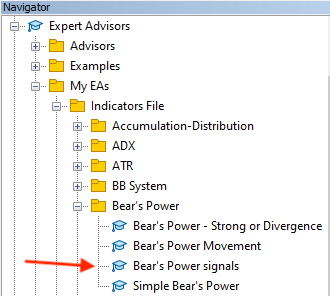By dragging and dropping this expert we will find the window of this expert the same as the following: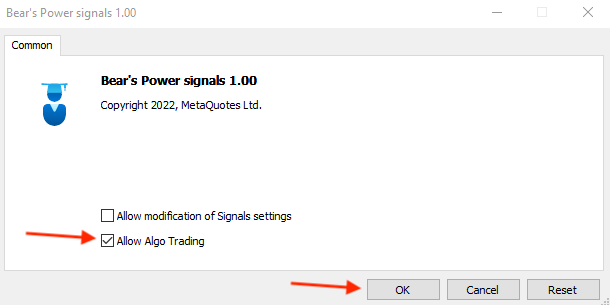After pressing "OK" we will find the expert attached to the chart the same as the following: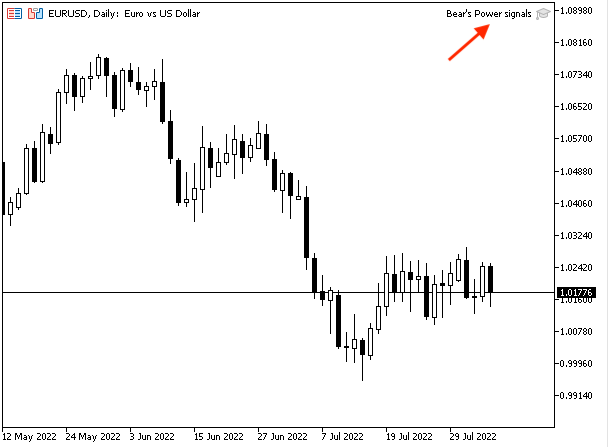We can see the expert is attached to the chart in the top right corner. Then, we can find generated signals based on this trading system the same as the following:

In case of the buy signal: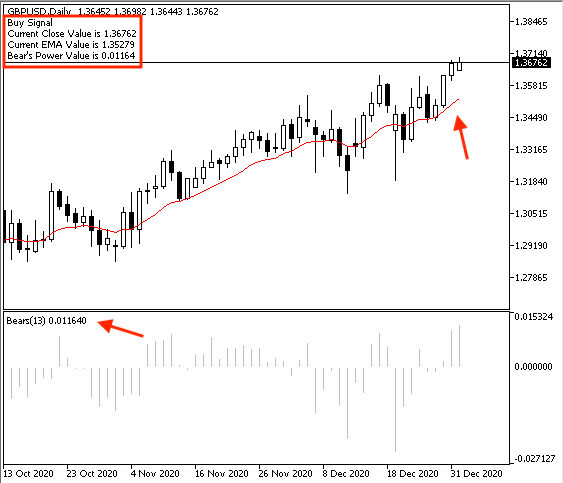As we can see on the chart we have a comment with the following values:

• Current close value
• Current EMA value
• Bear's Power value

In case of sell signal: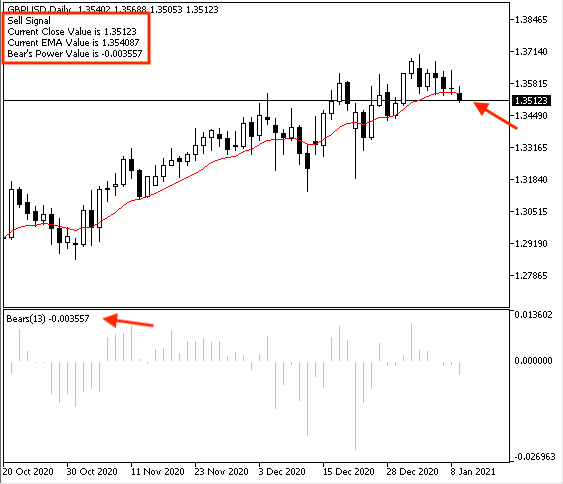As we can see on the chart the desired signal as a comment on the chart with the following values:

• Sell signal
• Current close value
• Current EMA value
• Bear's Power value

### Conclusion

Now, I think that we covered the basics of this indicator through the previous topics. We learned in more detail what is the Bear's Power indicator, what it measures, how we can calculate it, and how we can insert and use the MetaTrader 5 built-in Bear's Power indicator through the topic of Bear's Power definition. After understanding the indicator in detail, we learned how to use the indicator based on the basic idea behind the indicator through some mentioned simple strategies the basic objective of these strategies is to learn how we can use this indicator and you have to test them before using them by your real account to make sure that they are suitable for you and you can generate profits by them. These strategies were the same as the following:

Bear's Power Movement: this strategy can be used to determine the direction of the Bear's Power indicator if it is rising or declining.

Bear's Power Strong or divergence: this strategy can be used to determine if we have a strong move or divergence.

Bear's Power signals: this strategy can be used to get buy or sell signals.

After that, we learned how to design a step-by-step blueprint for every mentioned strategy to help us to create a trading system smoothly and easily after organizing our ideas through these blueprints. Then, we learn the most interesting topic in this article which was creating a trading system for every mentioned strategy to get signals automatically after coding them on MQL5 to be executed on the MetaTrader 5 trading terminal.

I hope that you applied and tried to write mentioned code by yourself as this will help you to improve your coding and learning process if you want and this will enhance improving your learning curve as a programmer. As we need to pay attention to code as much as we can and try to solve problems as much as we can also if we need to improve our programming skills. Programming is an important skill that we need to improve to help us to trade smoothly, easily, effectively, and accurately. It is also saving our time to do what we usually do in specific and determined steps, the computer or the program can do that in our favor automatically and also this approach can help us to avoid emotions which can be very harmful when it affects our trading negatively as the program will do what we need without any emotions.

I hope also that you find this article useful for you to enhance your trading and improve your trading results. If you want to read more similar articles about learning how to design a trading system based on the most popular technical indicators you can read my other articles in this series as we have many technical indicators that we shared in these articles and designed trading systems based on them and based on the basic concept behind them through simple strategies. You can find many of the most popular technical indicators in these articles for example moving average, MACD, Stochastic, RSI, and ADX.

Attached files |

#### Other articles by this author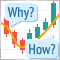Learn how to design a trading system by Bull's Power
Welcome to a new article in our series about learning how to design a trading system by the most popular technical indicator as we will learn in this article about a new technical indicator and how we can design a trading system by it and this indicator is the Bull's Power indicator.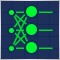Data Science and Machine Learning — Neural Network (Part 01): Feed Forward Neural Network demystified
Many people love them but a few understand the whole operations behind Neural Networks. In this article I will try to explain everything that goes behind closed doors of a feed-forward multi-layer perception in plain English.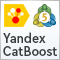Metamodels in machine learning and trading: Original timing of trading orders
Metamodels in machine learning: Auto creation of trading systems with little or no human intervention — The model decides when and how to trade on its own.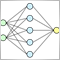Experiments with neural networks (Part 1): Revisiting geometry
In this article, I will use experimentation and non-standard approaches to develop a profitable trading system and check whether neural networks can be of any help for traders.